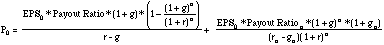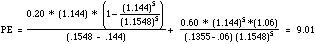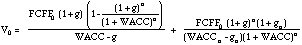Corrections to Valuation Book (in bold)

 Page Number Correction/Addendum 195 In illustration 10.2, Dividends per share in 1994 = \$ 2.00 (not \$ 2.04) 198 Fifth line from the top.. "the fundamental growth model described in Chapter 7 (not chapter 5). 200 In illustration 10.5 Length of the high-growth period = 5 years 219 FCFE = Net Income - (1 - Debt Ratio) (Capital expenditures - depreciation) - (1 - Debt ratio) (Change in Working Capital) {not added, but subtracted) 221 In illustration 11.1 Chg in Working Capital (instead of ¶ Working Capital) 231 The expected cash flows were computed using - (Cap Ex - Depr.) (.9) [not (Cap Ex - Depr.) (.09)] - Chg in Working Capital (.9) [not Chg in Working Capital (.09)] 238 The table has to be corrected as follows: EBITDA = FCFF + EBIT(t) + Capital Expenditures + Chg in Working Capital NOI (1-t) = FCFF + (Cap Ex - Depr) + Non oper Exp (1-t) + Chg in Working Capital EBIT (1-t) = FCFF + (Cap Ex - Depr) + Chg in Working Capital At the bottom of the table, EBIT (1-t) = EBITDA(1-t) - Depreciation (1-t) EBIT = NOI - Nonoperating expenses 243 Add to Base year information Market Value of debt outstanding now = \$ 2,740.58 million 255 The current FCFF is estimated by assuming that the change in working capital = 0 294295298 In illustration 14.6 The treasury bond rate used is 6% 310The same correction applies to the value/FCFF multiple. 340 See correction from page 294 above.